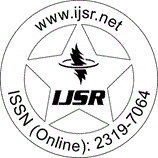International Journal of Science and Research (IJSR)
Call for Papers | Fully Refereed | Open Access | Double Blind Peer Reviewed

Downloads: 1 | Views: 41 | Weekly Hits: ⮙1 | Monthly Hits: ⮙1

Research Paper | Mathematics | India | Volume 11 Issue 4, April 2022

# Total Graph of Z_n and It's Adjacency Matrix

Pinku Sarkar | Kuntala Patra 

Abstract: Let G be a simple undirected graph. For a commutative ring R, Z(R)be the set of zero divisors of R. Total graph of R is denoted by T(?(R)), all elements of R are taken as vertices of the graph.Two distinct vertices a,b are adjacent if and only if a+b?Z(R). Here we study total graph T(?(R)) for R=Z_n. Also discuss degree, planarity, Eulerian property for different n. We also study adjacency matrix of the graph.

Keywords: Total Graph, Planarity, Adjacency matrix

Edition: Volume 11 Issue 4, April 2022,

Pages: 1017 - 1019